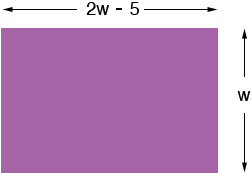SEARCH HOMEMath Central Quandaries & QueriesQuestion from tara, a student: The length if a rectangle is 5 feet less than twice its width. The perimeter of the rectangle is 38 feet. let w represent the width. write an equation for the perimeter of the rectangle in terms of w. Then solve the equation to find the length and width of the rectangle.Hi Tara,

Draw a diagram.I labeled the width w feet since the problem said the width was w. I labeled the length 2w - 5 since the problem said that the length was 5 feet less than twice the width. Looking at the diagram, what is the perimeter of the rectangle in terms of w? This expression is equal to 38 feet. Solve for w.

PennyMath Central is supported by the University of Regina and The Pacific Institute for the Mathematical Sciences.